Question

1. A 0.750-g sample of tin metal reacts with 0.201 g of oxygen gas to form tin oxide.

1. A 0.750-g sample of tin metal reacts with 0.201 g of oxygen gas to form tin oxide. Calculate the empirical formula of the tin oxide.

2. A 0.565-g sample of cobalt metal reacts with excess sulfur to give 1.027 g of cobalt sulfide. Calculate the empirical formula of the product.

3. A 1.164-g sample of iron filings reacts with chlorine gas to give 3.384 g of iron chloride. Calculate the empirical formula of the product?

4. A 0.626-8 sample of copper oxide was reduced to 0.500 g of copper metal by heating in a stream of hydrogen gas. Calculate the empirical formula of the copper oxide.

5. A sample of phosphorus weighing 0.500 g was ignited to the oxide in a stream of oxygen gas. What is the empirical formula of phosphorus oxide if the product has a mass of 1.145 7 What is the molecular formula of phosphorus oxide if the molar mass is approximately 285 pmol?

6. (optional) Ethylene glycol, the main ingredient in antifreeze, contains 38.7% carbon 9.7% hydrogen, and 51.6% oxygen. Calculate the empirical and molecular formulas for ethylene glycol, given the molar mass is approximately 60 g/mol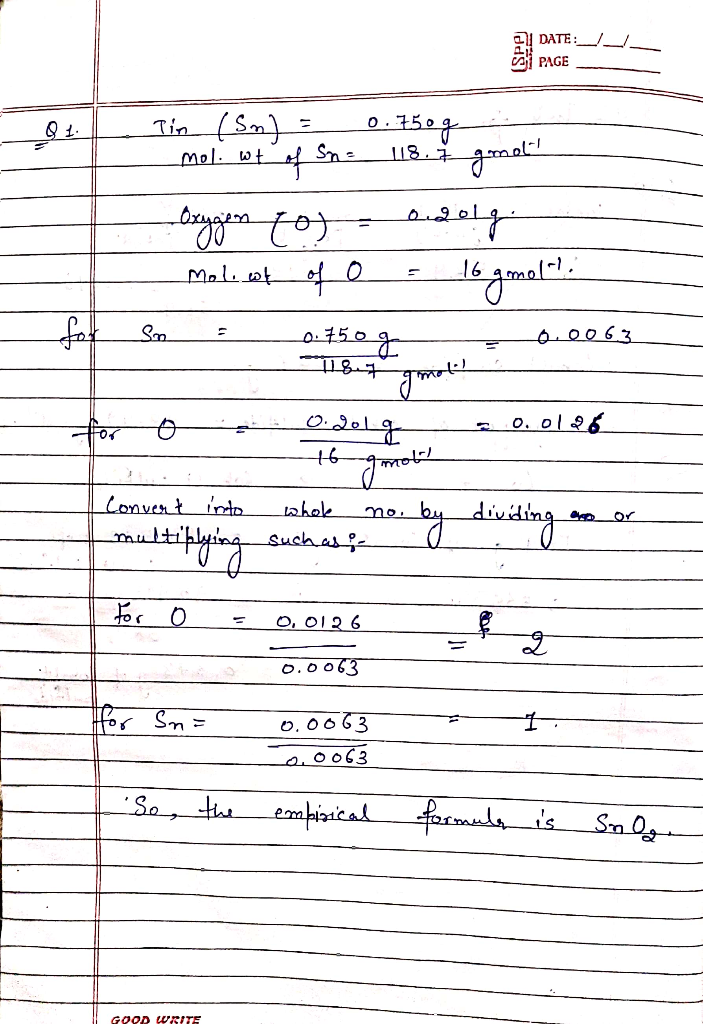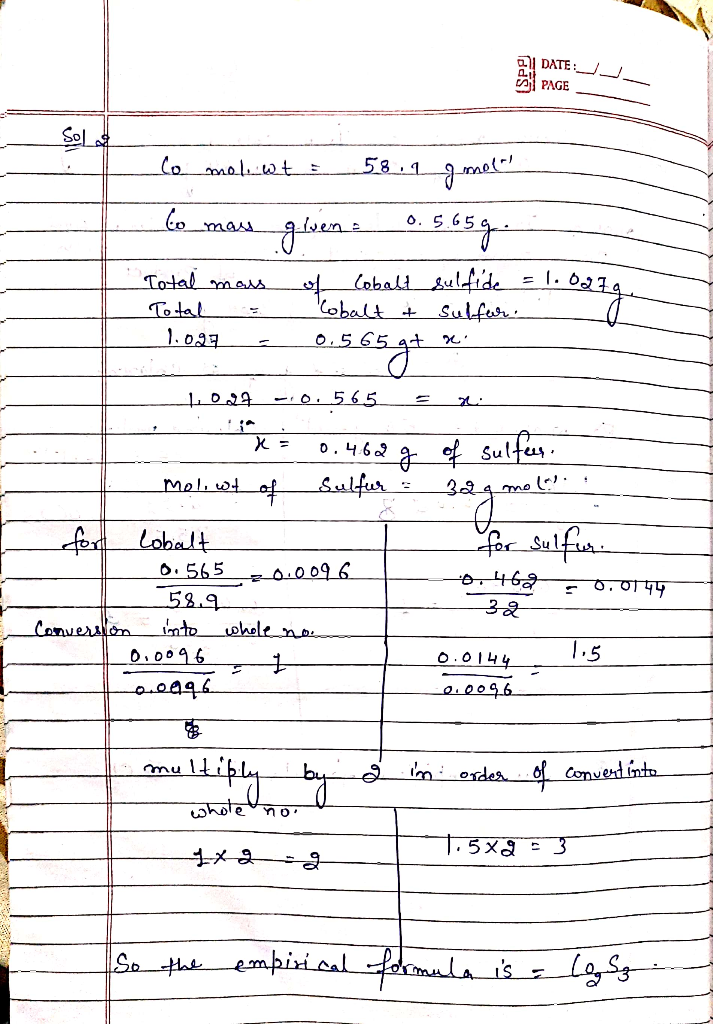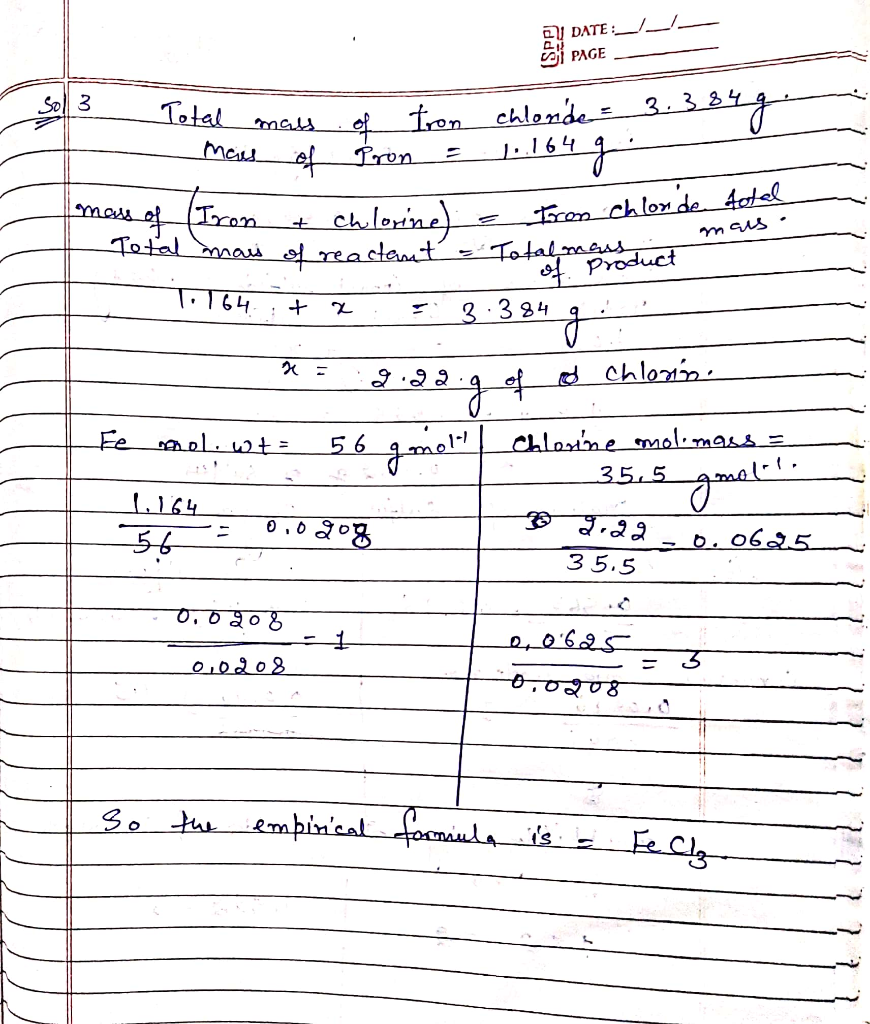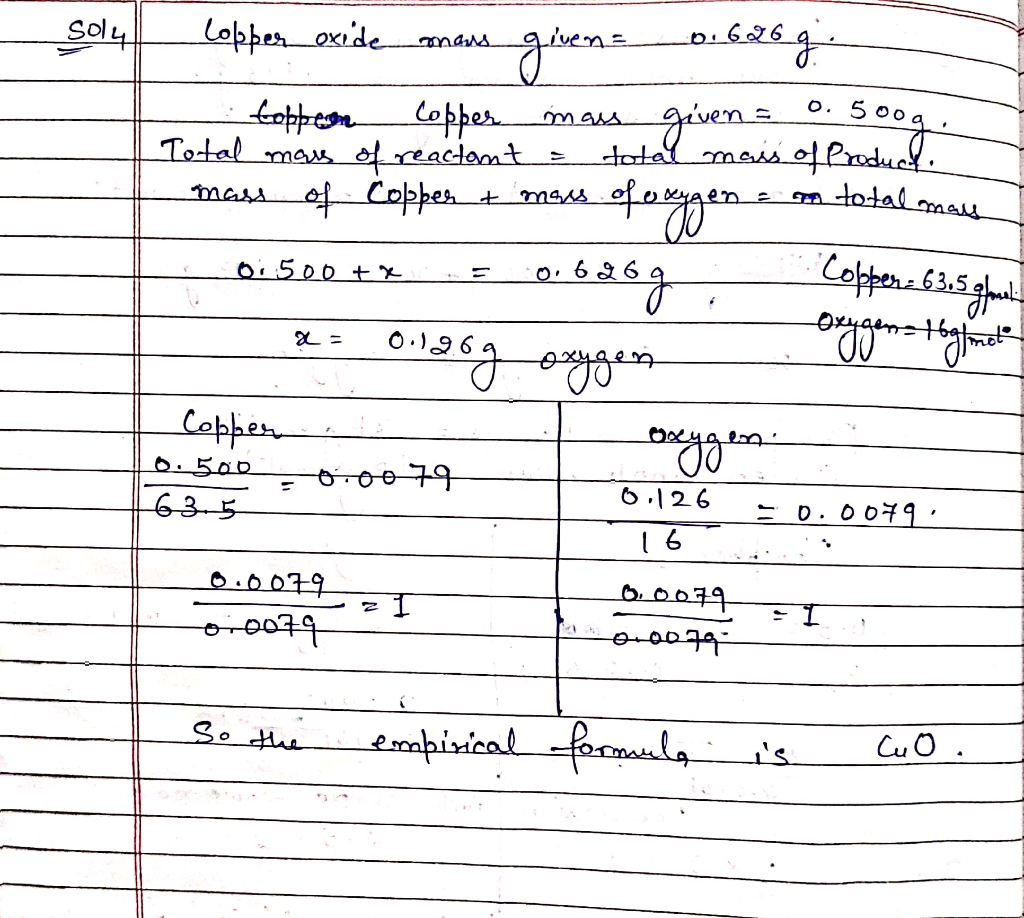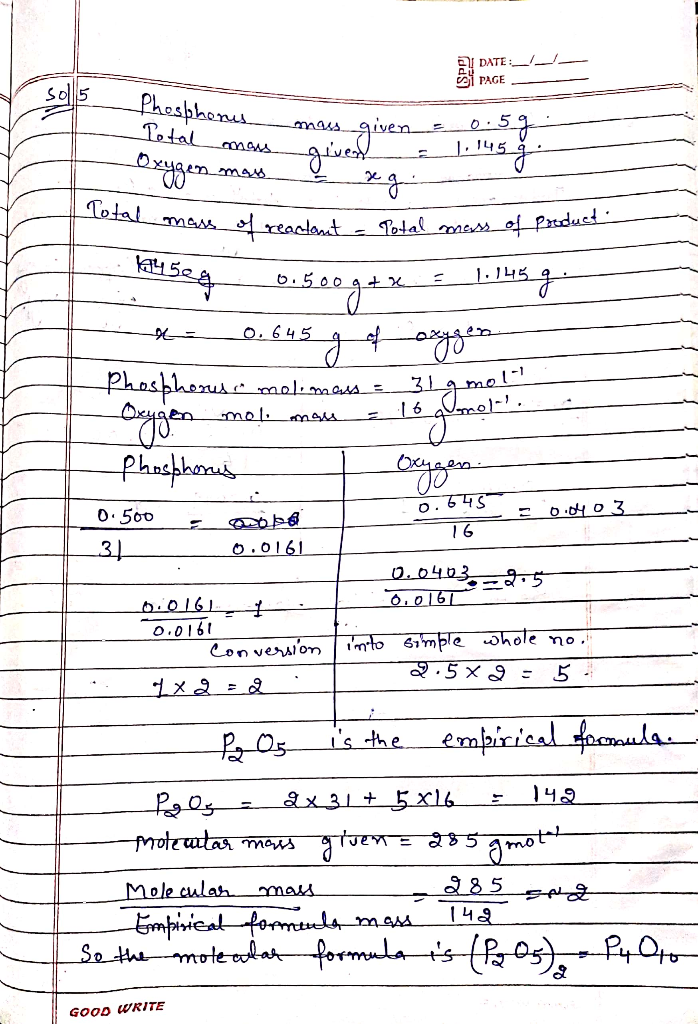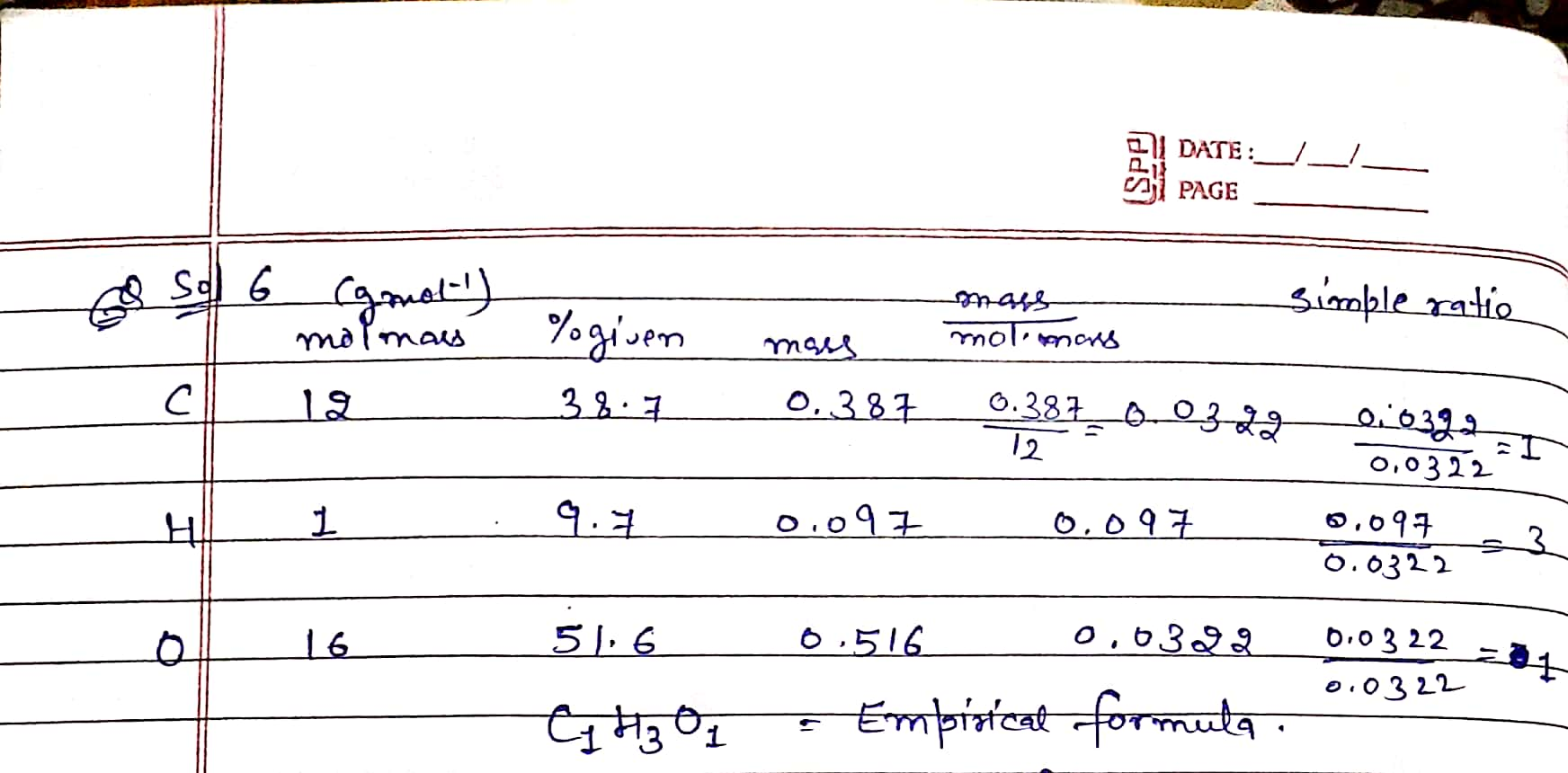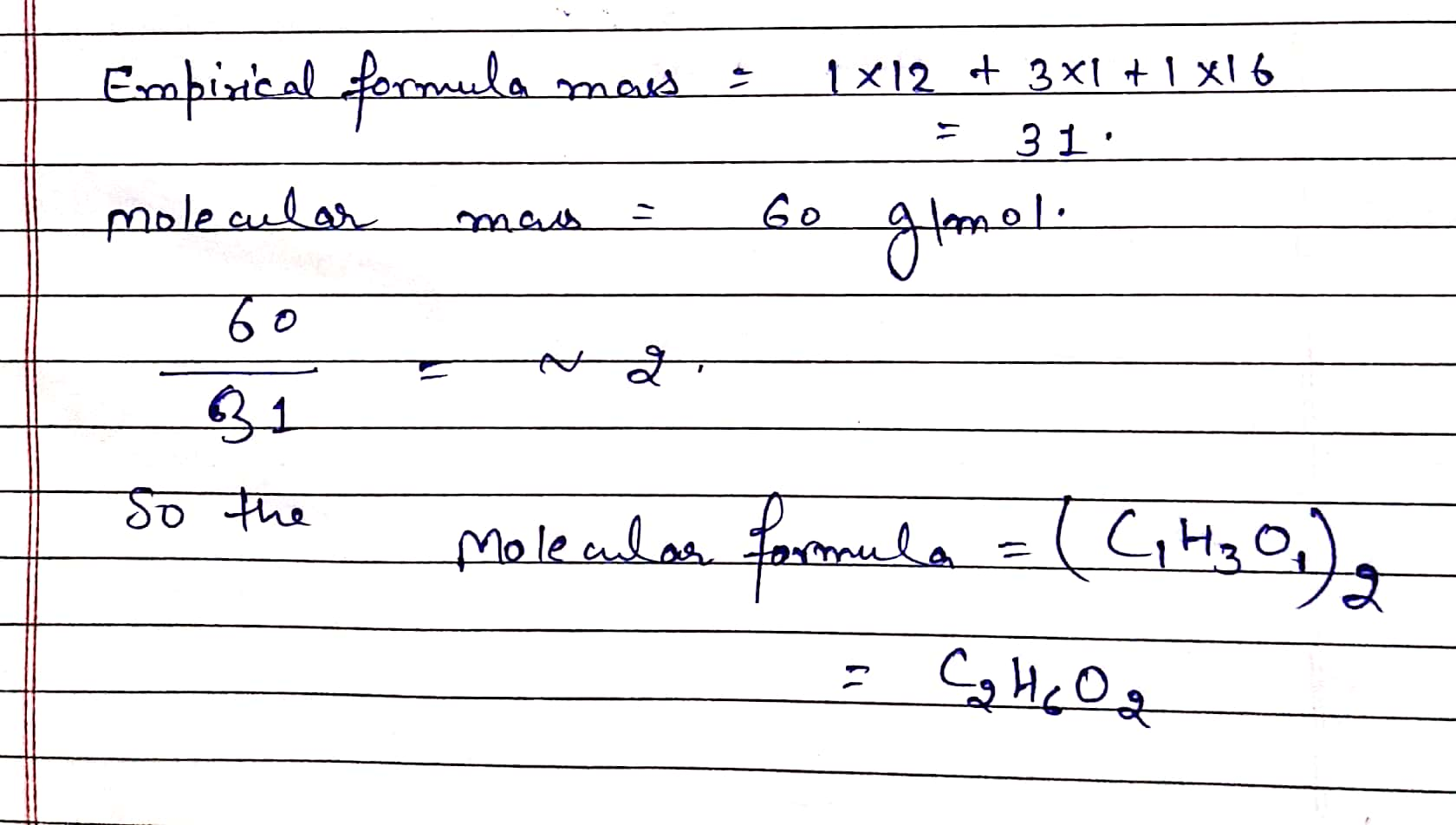Earn Coins

Coins can be redeemed for fabulous gifts.

Similar Homework Help Questions
• 5. A 0.565-8 sample of cobalt metal reacted with excess sulfur powder to give 1.027 g of cobalt sulfide

5. A 0.565-8 sample of cobalt metal reacted with excess sulfur powder to give 1.027 g of cobalt sulfide Calculate the empirical formula of the product. 6. A 0.750-g sample of tin foil was heated in air and reacted with oxygen gas to give 0.953 g of tin oxide. Calculate the empirical formula of the product. 7. (optional) A 1.000-g sample of red phosphorus powder was burned in air and reacted with oxygen gas to give 2.291 g of phosphorus oxide. Calculate...

• 1. When 2.50 g of copper metal reacts with molecular oxygen, the copper oxide product of the reaction has a mass of...

1. When 2.50 g of copper metal reacts with molecular oxygen, the copper oxide product of the reaction has a mass of 2.81 g. What is the empirical formula of the copper oxide product 2. When 10.8 g of silver was reacted with sulfur. 12.4 grams of product was produced there was only one product). What is the empirical formula of the product? 3. Lysine is a compound composed of carbon, hydrogen, nitrogen and oxygen. When 1.50 g of lysine is burned,...

• 40.505-8 sample of cobalt metal reacted with excess sulfur powder to give 1.027 g of cobalt...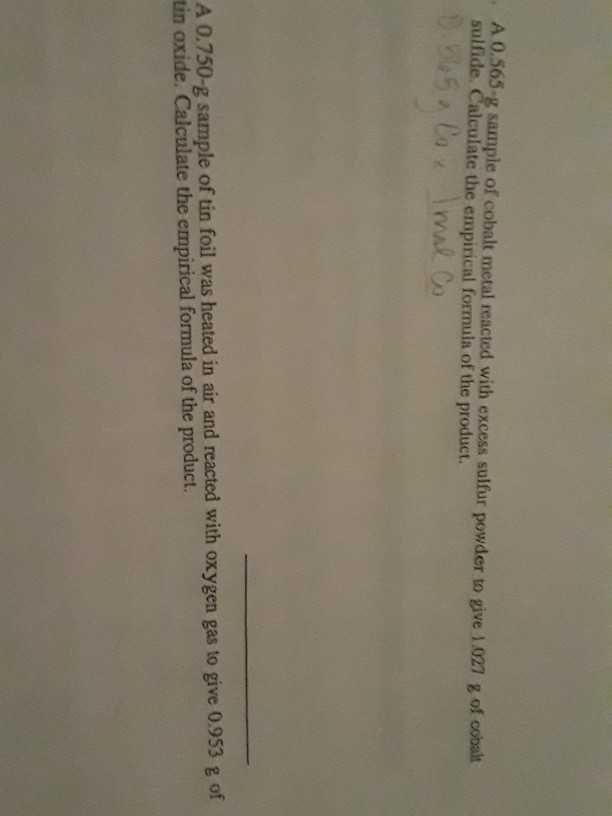40.505-8 sample of cobalt metal reacted with excess sulfur powder to give 1.027 g of cobalt sulfide. Calculate the empirical formula of the product. D. 565. Cox Imal Co A 0.750-g sample of tin foil was heated in air and reacted with oxygen gas to give 0.953 g of tin oxide. Calculate the empirical formula of the product.

• A 1.500 g piece of calcium metal reacts with oxygen gas to form 2.099 g of...

A 1.500 g piece of calcium metal reacts with oxygen gas to form 2.099 g of calcium oxide. Calculate the empirical formula of calcium oxide

• write clearly d. 0.626 g sample of copper oxide was reduced to 0.500 g of copper...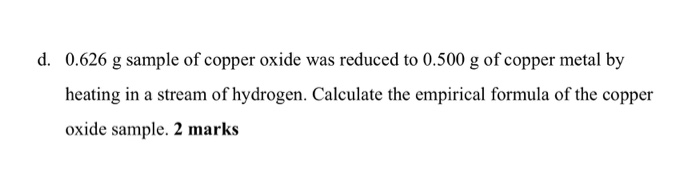write clearly d. 0.626 g sample of copper oxide was reduced to 0.500 g of copper metal by heating in a stream of hydrogen. Calculate the empirical formula of the copper oxide sample. 2 marks

• a 1.000g sample of red phosphorus powder was burned in air and.reacted with oxygen gas to give...

a 1.000g sample of red phosphorus powder was burned in air and.reacted with oxygen gas to give 2.291g of phosphorus oxide. calculate the empirical formula andmolecular formula of phosphorus oxide give the molar mass is approximately 282g/mol?

• A 43.29 gram sample of cobalt is heated in the presence of excess oxygen. A metal...

A 43.29 gram sample of cobalt is heated in the presence of excess oxygen. A metal oxide is formed with a mass of 60.92 g. Determine the empirical formula of the metal oxide.

• A 21.92 gram sample of iron is heated in the presence of excess oxygen. A metal...

A 21.92 gram sample of iron is heated in the presence of excess oxygen. A metal oxide is formed with a mass of 31.33 g. Determine the empirical formula of the metal oxide. A 25.23 gram sample of chromium is heated in the presence of excess fluorine. A metal fluoride is formed with a mass of 43.67 g. Determine the empirical formula of the metal fluoride. A 7.199 gram sample of iron is heated in the presence of excess bromine....

• A 50.63 gram sample of cobalt is heated in the presence of excess oxygen. A metal...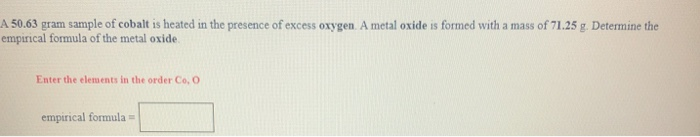A 50.63 gram sample of cobalt is heated in the presence of excess oxygen. A metal oxide is formed with a mass of 71.25 g. Determine the empirical formula of the metal oxide Enter the elements in the order Co, O empirical formular

• a sample of titanium with a mass of 6.48g reacts with oxygen to form 10.80 g...

a sample of titanium with a mass of 6.48g reacts with oxygen to form 10.80 g of the metal oxide. determine the emperical formula of the metal oxide